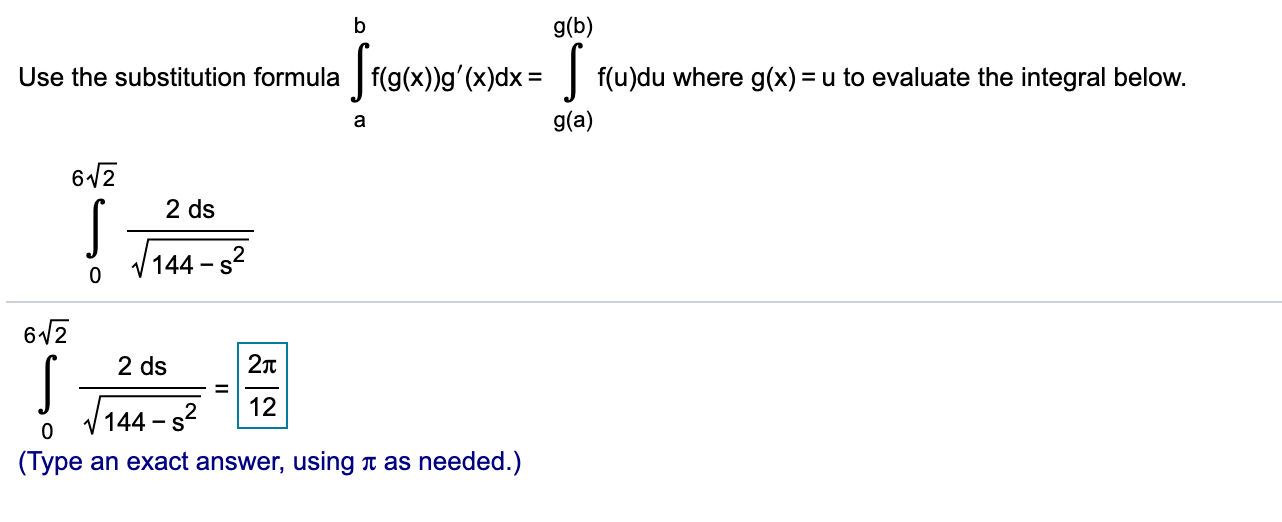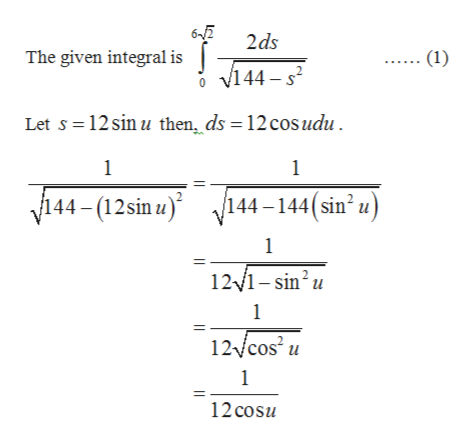# g(b)Use the substitution formula f(g(x))g'(x)dxf(u)du where g(x) = u to evaluate the integral belowg(a)a6122 dsV144 s206122Tt2 ds12V144 2-S0(Туреas needed.)an exact answer,

Question
9 views

Use the substitution formula

Integral from a to b f left parenthesis g left parenthesis x right parenthesis right parenthesis g prime left parenthesis x right parenthesis dx

equalsIntegral from g left parenthesis a right parenthesis to g left parenthesis b right parenthesis f left parenthesis u right parenthesis du

where

​g(x)equals
u

to evaluate the integral below.help_outlineImage Transcriptioncloseg(b) Use the substitution formula f(g(x))g'(x)dx f(u)du where g(x) = u to evaluate the integral below g(a) a 612 2 ds V144 s2 0 612 2Tt 2 ds 12 V144 2 -S 0 (Туре as needed.) an exact answer, fullscreen
check_circle

Step 1

Obtain the value of the integral as follows.help_outlineImage Transcriptionclose62 The given integral is J J144- s2 2ds (1) Let s 12 sin u then ds 12 cosudu 1 1 144-(12sin u144-144(sin* u) 1 12/1-sin2u 1 12/cos u 1 12 cosu fullscreen
Step 2

Substitute the obtained values in equation (1),

Step 3

Compute the limit ...

### Want to see the full answer?

See Solution

#### Want to see this answer and more?

Solutions are written by subject experts who are available 24/7. Questions are typically answered within 1 hour.*

See Solution
*Response times may vary by subject and question.
Tagged in

### Integration